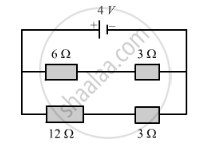Share

Books Shortlist

# For the Circuit Shown in the Diagram Below: - CBSE Class 10 - Science

ConceptElectric Potential Difference

#### Question

For the circuit shown in the diagram below:What is the value of:

(i) current through 6 Ω resistor?
(ii) potential difference across 12 Ω resistor?

#### Solution

The resistors of 6 Ω and 3 Ω are connected in series. Therefore, their net resistance can be calculated as:
R = R1 + R2
Here, R1 = 6 Ω
R2 = 3 Ω
So:
R = 6 Ω + 3 Ω = 9 Ω
The current through this branch, I = V/R
I = 4/9 = 0.44 A
In a series combination, the current remains the same. So the current through the 6 Ω resistor is 0.44 A.

(2) The current through the branch with resistors of 12 Ω and 3 Ω:
I = V/R
I = 4/(12 + 3) = 4 / 15 A
The potential difference across the 12 Ω resistance can be obtained by using the equation,
V = IR.
V = (4 / 15) x 12 = 3.2 V

Is there an error in this question or solution?

#### Video TutorialsVIEW ALL 

Solution For the Circuit Shown in the Diagram Below: Concept: Electric Potential Difference.
S